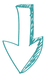top of page

## beautiful MODELS

Gravity is designed in a way you can write elegant, compact models.

First, you will need an IDE, I recommend using one of the following:## Constants, Parameters and Variables

Parameters and variables inherit from the constant class. The type and the numerical precision of a constant can be set by the user. Currently supported types are presented in Code Block 1.Parameters are used to represent named constants, i.e., input data that is assigned a name and a set of values.
The same types presented in Code Block 1 apply for parameters. Code Block 2 presents examples on declaring different parameters and assigning corresponding values.Finally, Code Block 3 illustrates the declaration of variables, which are treated as bounded parameters.## Functions and Constraints

In order to exploit structure, the building blocks of a function are split into four different parts.
A function has a linear part, a quadratic part, a polynomial part, and an expression tree as depicted in equation 1.where a^T denotes the transpose of the coefficient vector appearing in the linear part, Q represents the quadratic coefficients matrix, P denotes the polynomial part and E represents the expression tree gathering the remaining nonlinear terms in f. E(x) can represent either unary or binary expressions, unary operators currently supported in Gravity include exp, log, sqrt, sin, and cos. Binary operators include multiplication, addition, subtraction, division, and power.
Depending on the function type, the corresponding part will be populated.
A constraint is a function with a right-hand-side and an operator that can take values from <=, >=, and =.
Constraint examples are given in Code Block 4.Gravity can recognize convexity preserving operations and implements a set of sufficient conditions for checking if a function is convex, concave or undetermined. Code Block 5 illustrates this feature on a quadratic constraint.## Abstract Models: Template Constraints

Template constraints can help reduce computational time for building the model and for operations that only require the symbolic structure of the underlying functions, e.g., computing derivatives and detecting convexity. Code Block 6 showcases template constraints and the way Gravity handles their indexing.

Graph aware. Gravity has an underlying graph implementation and indexing can be done on nodes, edges, and node pairs (pairs of nodes joined by one or more edges) of the graph as shown in Code Block 6. Some useful graph algorithms such as tree decomposition and cycle basis computation are also implemented in Gravity.Simple Graph

n1

n2

Lazy Constraint Generation. Gravity can add both linear and nonlinear constraints in a lazy fashion.

If you use the function add_lazy(Constraint), then all the instances of the template constraint are only added if they are violated, in an iterative fashion.

## Models and Solvers

A model is a collection of variables, constraints and an objective function. Populating a model can be done by invoking the function presented in Code Block 7.Gravity currently links to a small number of solvers including Cplex, Gurobi, Ipopt, Bonmin, and Mosek. We plan to support more solvers such as Scip, XpressMP, Couenne and SAT solvers such as MiniSat in the near future. The way to invoke a solver is illustrated in Code Block 8.# Interested in more advanced examples?Example 1. Mixed-Integer Linear Optimization: Stable Set Problem

Example 2. Nonlinear Optimization:

Power Systems Optimization

bottom of page